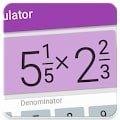# Fraction calculator: easy solve fractions problems APK

Best math calculator with fractions, decimals, percentages, and parentheses that shows a detailed solution for all calculations.Perfect for students, parents, teachers and woodworkers to solve or verify fraction problems.

At school or home need to resolve simple or complex fraction problems convert fractions into decimals or decimals into fractions? – this app will do it and will show how it was done

Features of this app

Supports combining decimal and fractional numbers. Automatically reduces fraction result to its simplest form.

Converts fractions into decimals and decimals into fractions. Calculated result is displayed using fractions. Equivalent value in decimals is also available.

Shows detailed solution, step by step for fraction problems and calculations.

History of previous calculations is available.

Besides basic math operations such as: add, subtract, multiply and divide; app allows calculating percentages for fractions.

Works with big numbers. No limits

We’ve made this fraction calculator app available in multiple colors for you!

#### What’s New

Minor UI improvements.